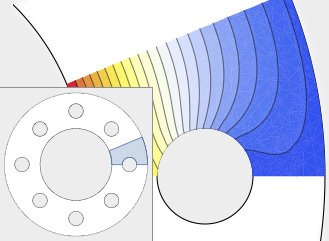# PDEs and Finite Elements

Version 10 extends its numerical differential equation-solving capabilities to include the finite element method. Given a PDE, a domain, and boundary conditions, the finite element solution processincluding grid and element generationis fully automated. Stationary and transient solutions to a single PDE or a system of partial differential equations are supported for one, two, and three dimensions.• Solve partial differential equations over arbitrarily shaped regions. »
• Solve stationary and transient PDEs in one, two, and three dimensions.
• Solve coupled systems of PDEs.
• Specify Dirichlet boundary conditions. »
• Specify generalized Neumann and Robin values. »
• Support for linear PDEs with coefficients that are variable in time and space.
• Freely formulate coupled PDEs for multiphysics analysis.
• Specify domains using the full geometric region framework.
• Fully automatic mesh generation from any region.
• Obtain solutions as approximate functions that can be further analyzed.
• Full suite of intermediate-level data structures and solution functions for detailed control and analysis of the solution. »
• Support for explicit mesh generation.
• Support for meshes with first- and second-order interpolation.
• Support for meshes with curved boundaries.

## Mathematica + Mathematica Online

Questions? Comments? Contact a Wolfram expert »